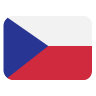3.4Sections# Images

Phalcon\Image is the component that allows you to manipulate image files. Multiple operations can be performed on the same image object.

This component makes use of adapters to encapsulate specific image manipulator programs. The following image manipulator programs are supported:

Class Description
Phalcon\Image\Adapter\Gd Requires the GD PHP extension
Phalcon\Image\Adapter\Imagick Requires the ImageMagick PHP extension

### Factory

Loads an Image Adapter class using `adapter` option.

``````<?php

use Phalcon\Image\Factory;

\$options = [
'width'   => 200,
'height'  => 200,
];

``````

The Phalcon\Image\AdapterInterface interface must be implemented in order to create your own image adapters or extend the existing ones.

## Saving and rendering images

Before we begin with the various features of the image component, it’s worth understanding how to save and render these images.

``````<?php

\$image = new \Phalcon\Image\Adapter\Gd('image.jpg');

// ...

// Overwrite the original image
\$image->save();
``````
``````<?php

\$image = new \Phalcon\Image\Adapter\Gd('image.jpg');

// ...

// Save to 'new-image.jpg'
\$image->save('new-image.jpg');
``````

You can also change the format of the image:

``````<?php

\$image = new \Phalcon\Image\Adapter\Gd('image.jpg');

// ...

// Save as a PNG file
\$image->save('image.png');
``````

When saving as a JPEG, you can also specify the quality as the second parameter:

``````<?php

\$image = new \Phalcon\Image\Adapter\Gd('image.jpg');

// ...

// Save as a JPEG with 80% quality
\$image->save('image.jpg', 80);
``````

## Resizing images

There are several modes of resizing:

• `\Phalcon\Image::WIDTH`
• `\Phalcon\Image::HEIGHT`
• `\Phalcon\Image::NONE`
• `\Phalcon\Image::TENSILE`
• `\Phalcon\Image::AUTO`
• `\Phalcon\Image::INVERSE`
• `\Phalcon\Image::PRECISE`

### `\Phalcon\Image::WIDTH`

The height will automatically be generated to keep the proportions the same; if you specify a height, it will be ignored.

``````<?php

\$image = new \Phalcon\Image\Adapter\Gd('image.jpg');

\$image->resize(
300,
null,
\Phalcon\Image::WIDTH
);

\$image->save('resized-image.jpg');
``````

### `\Phalcon\Image::HEIGHT`

The width will automatically be generated to keep the proportions the same; if you specify a width, it will be ignored.

``````<?php

\$image = new \Phalcon\Image\Adapter\Gd('image.jpg');

\$image->resize(
null,
300,
\Phalcon\Image::HEIGHT
);

\$image->save('resized-image.jpg');
``````

### `\Phalcon\Image::NONE`

• The `NONE` constant ignores the original image’s ratio.
• Neither width and height are required.
• If a dimension is not specified, the original dimension will be used.
• If the new proportions differ from the original proportions, the image may be distorted and stretched.
``````<?php

\$image = new \Phalcon\Image\Adapter\Gd('image.jpg');

\$image->resize(
400,
200,
\Phalcon\Image::NONE
);

\$image->save('resized-image.jpg');
``````

### `\Phalcon\Image::TENSILE`

• Similar to the `NONE` constant, the `TENSILE` constant ignores the original image’s ratio.
• Both width and height are required.
• If the new proportions differ from the original proportions, the image may be distorted and stretched.
``````<?php

\$image = new \Phalcon\Image\Adapter\Gd('image.jpg');

\$image->resize(
400,
200,
\Phalcon\Image::TENSILE
);

\$image->save('resized-image.jpg');
``````

## Cropping images

For example, to get a 100px by 100px square from the centre of the image:

``````<?php

\$image = new \Phalcon\Image\Adapter\Gd('image.jpg');

\$width   = 100;
\$height  = 100;
\$offsetX = ((\$image->getWidth() - \$width) / 2);
\$offsetY = ((\$image->getHeight() - \$height) / 2);

\$image->crop(\$width, \$height, \$offsetX, \$offsetY);

\$image->save('cropped-image.jpg');
``````

## Rotating images

``````<?php

\$image = new \Phalcon\Image\Adapter\Gd('image.jpg');

// Rotate an image by 90 degrees clockwise
\$image->rotate(90);

\$image->save('rotated-image.jpg');
``````

## Flipping images

You can flip an image horizontally (using the `\Phalcon\Image::HORIZONTAL` constant) and vertically (using the `\Phalcon\Image::VERTICAL` constant):

``````<?php

\$image = new \Phalcon\Image\Adapter\Gd('image.jpg');

// Flip an image horizontally
\$image->flip(
\Phalcon\Image::HORIZONTAL
);

\$image->save('flipped-image.jpg');
``````

## Sharpening images

The `sharpen()` method takes a single parameter - an integer between 0 (no effect) and 100 (very sharp):

``````<?php

\$image = new \Phalcon\Image\Adapter\Gd('image.jpg');

\$image->sharpen(50);

\$image->save('sharpened-image.jpg');
``````

## Adding watermarks to images

``````<?php

\$image = new \Phalcon\Image\Adapter\Gd('image.jpg');

\$watermark = new \Phalcon\Image\Adapter\Gd('me.jpg');

// Put the watermark in the top left corner
\$offsetX = 10;
\$offsetY = 10;

\$opacity = 70;

\$image->watermark(
\$watermark,
\$offsetX,
\$offsetY,
\$opacity
);

\$image->save('watermarked-image.jpg');
``````

Of course, you can also manipulate the watermarked image before applying it to the main image:

``````<?php

\$image = new \Phalcon\Image\Adapter\Gd('image.jpg');

\$watermark = new \Phalcon\Image\Adapter\Gd('me.jpg');

\$watermark->resize(100, 100);
\$watermark->rotate(90);
\$watermark->sharpen(5);

// Put the watermark in the bottom right corner with a 10px margin
\$offsetX = (\$image->getWidth() - \$watermark->getWidth() - 10);
\$offsetY = (\$image->getHeight() - \$watermark->getHeight() - 10);

\$opacity = 70;

\$image->watermark(
\$watermark,
\$offsetX,
\$offsetY,
\$opacity
);

\$image->save('watermarked-image.jpg');
``````

## Blurring images

The `blur()` method takes a single parameter - an integer between 0 (no effect) and 100 (very blurry):

``````<?php

\$image = new \Phalcon\Image\Adapter\Gd('image.jpg');

\$image->blur(50);

\$image->save('blurred-image.jpg');
``````

## Pixelating images

The `pixelate()` method takes a single parameter - the higher the integer, the more pixelated the image becomes:

``````<?php

\$image = new \Phalcon\Image\Adapter\Gd('image.jpg');

\$image->pixelate(10);

\$image->save('pixelated-image.jpg');
``````